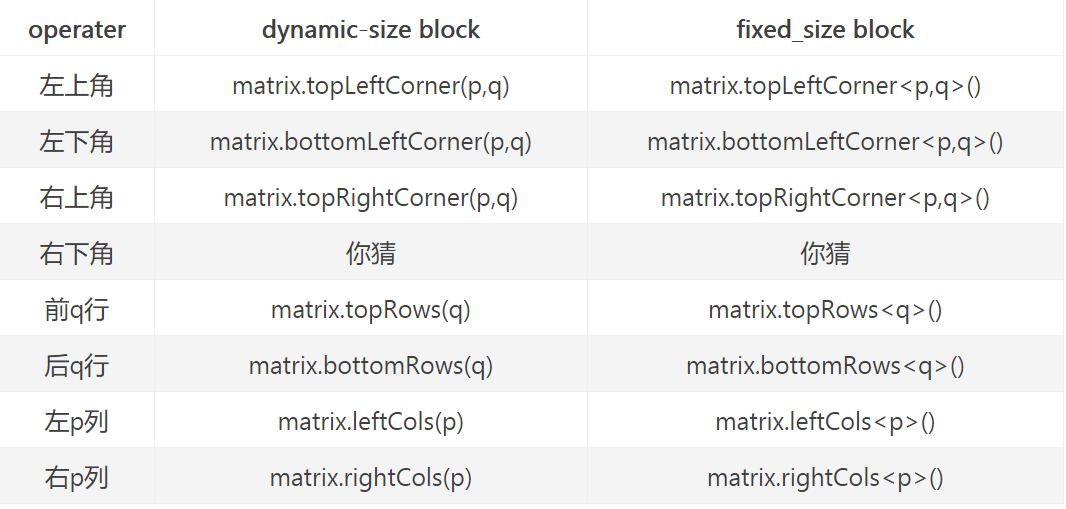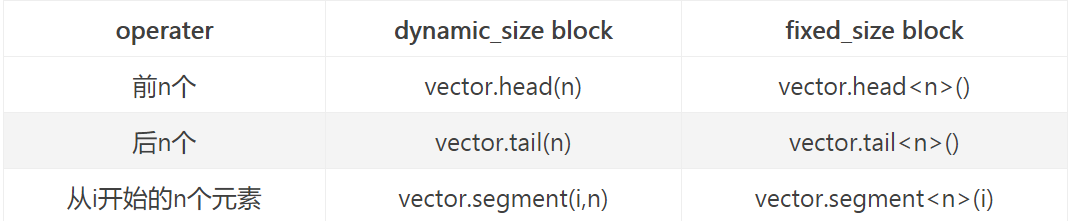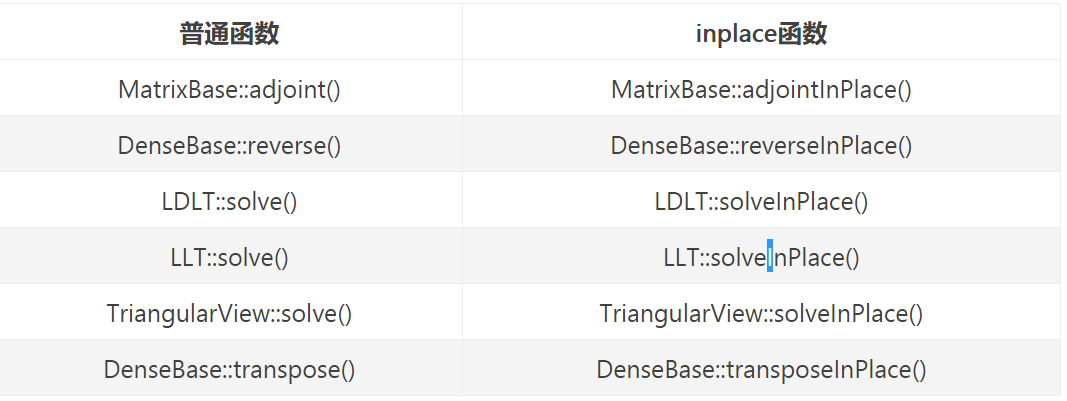• Eigen库使用汇总
2020-08-23 16:00:05

### 记录使用过的Eigen功能

2：使用eigen库进行空间变换 旋转平移，尺度缩放，放射变换

更多相关内容
• 当时使用Eigen库的目的是，将Matlab写的，LPCC和MFCC两种声音识别算法，十字形声阵列的MUSIC定位算法，和SVM分类器算法，转换成C++然后移植到到ARM处理器上（操作系统级上的并不是裸机）。而使用Eigen库的原因就是，...
• eigen3源码操作示例程序vs2015程序示例,带说明文档，保证通俗易懂，迅速走入eigen开发大门
• Eigen库使用指南(转载) 目录 Eigen库使用指南(转载) 1.模块和头文件 2. Matrix类 3. 矩阵与向量的运算 4. Array类 5. 块操作 6. 矩阵初始化 7. 归约，迭代器，广播 8. Map类 9. 混淆问题 1.模块和头文件...

# Eigen库使用指南(转载)

目录

Eigen库使用指南(转载)

1.模块和头文件

2. Matrix类

3. 矩阵与向量的运算

4. Array类

5. 块操作

6. 矩阵初始化

7. 归约，迭代器，广播

8. Map类

9. 混淆问题

## 1.模块和头文件

• Core #include<Eigen/Core>，包含MatrixArray类，基础的线性代数运算数组操作。
• Geometry #include<Eigen/Geometry>，包含旋转，平移，缩放，2维和3维的各种变换
• LU #include<Eigen/LU>，包含求逆，行列式，LU分解
• Cholesky #include<Eigen/Cholesky>，包含LLT和LDLT Cholesky分解
• SVD #include<Eigen/SVD>，包含SVD分解
• QR #include<Eigen/QR>，包含QR分解
• Eigenvalues #include<Eigen/Eigenvalues>，包含特征值，特征向量分解
• Sparse #include<Eigen/Sparse>，包含稀疏矩阵的存储和运算
• Dense #include<Eigen/Dense>，包含了Core/Geometry/LU/Cholesky/SVD/QR/Eigenvalues模块
• Eigen #include<Eigen/Eigen>，包含Dense和Sparse

## 2. Matrix类

• 所有矩阵和向量都是Matrix模板类的对象Matrix类有6个模板参数，主要使用前三个，剩下的使用默认值。
Matrix<typename Scalar,
int RowsAtCompileTime,
int ColsAtCompileTime,
int Options = 0,
int MaxRowsAtCompileTime = RowsAtCompileTime,
int MaxColsAtCompileTime = ColsAtCompileTime>
# Scalar 元素类型
# RowsAtCompileTime 行
# ColsAtCompileTime 列
# 例 typedef Matrix<int, 3, 3> Matrix3i;
# Options 比特标志位
# MaxRowsAtCompileTime和MaxColsAtCompileTime表示在编译阶段矩阵的上限。

# 列向量
typedef Matrix<double, 3, 1> Vector3d;
# 行向量
typedef Matrix<float, 1, 3> RowVector3f;

# 动态大小
typedef Matrix<double, Dynamic, Dynamic> MatrixXd;
typedef Matrix<float, Dynamic, 1> VectorXf;

• 默认构造时，指定大小的矩阵，只分配相应大小的空间，不进行初始化动态大小的矩阵，则未分配空间
• []操作符可以用于向量元素的获取，但不能用于matrix。Eigen支持以下的读/写元素语法：
matrix(i,j);
vector(i)
vector[i]
vector.x() // first coefficient
vector.y() // second coefficient
vector.z() // third coefficient
vector.w() // fourth coefficient
• matrix的大小可以通过rows(), cols(), size()获取，resize()可以重新调整矩阵大小。
• 上述的元素访问方法都通过断言检查范围，代价比较大。
1.  通过定义EIGEN_NO_DEBUG 或 NDEBUG，取消断言。
2.  通过使用coeff()和coeffRef()，来取消检查。比如，MatrixBase::coeff(int,int) const, MatrixBase::coeffRef(int,int)等。

## 3. 矩阵与向量的运算

• Eigen不支持类型自动转化，因此矩阵元素类型必须相同
• 支持+, -, +=, -=, *, /, *=, /=基础四则运算。
• 转置和共轭
MatrixXcf a = MatrixXcf::Random(3,3);
a.transpose();  # 转置
a.conjugate();  # 共轭
a.transposeInPlace() #原地转置

Vector3d v(1,2,3);
Vector3d w(4,5,6);
v.dot(w);    # 点积
v.cross(w);  # 叉积

Matrix2d a;
a << 1, 2, 3, 4;
a.sum();      # 所有元素求和
a.prod();      # 所有元素乘积
a.mean();    # 所有元素求平均
a.minCoeff();    # 所有元素中最小元素
a.maxCoeff();   # 所有元素中最大元素
a.trace();      # 迹，对角元素的和
# minCoeff和maxCoeff还可以返回结果元素的位置信息
int i, j;
a.minCoeff(&i, &j);

## 4. Array类

• Array是个类模板，前三个参数必须指定，后三个参数可选。
Array<typename Scalar,
int RowsAtCompileTime,
int ColsAtCompileTime>
# 常见类定义
typedef Array<float, Dynamic, 1> ArrayXf
typedef Array<float, 3, 1> Array3f
typedef Array<double, Dynamic, Dynamic> ArrayXXd
typedef Array<double, 3, 3> Array33d

ArrayXf a = ArrayXf::Random(5);
a.abs();    # 绝对值
a.sqrt();    # 平方根
a.min(a.abs().sqrt());  # 两个array相应元素的最小值
• 当执行array*array时，执行的是相应元素的乘积，所以两个array必须具有相同的尺寸。
• Matrix对象——>Array对象：.array()函数
• Array对象——>Matrix对象：.matrix()函数

## 5. 块操作

块是matrixarray中的矩形子块。

// 方法1
.block(i, j, p, q)    //起点(i, j)，块大小(p, q)，构建一个动态尺寸的block
.block<p, q>(i, j)  // 构建一个固定尺寸的block
• matrix.row(i): 矩阵第i行
• matrix.col(j): 矩阵第j列
• 角相关操作• Vector的块操作## 6. 矩阵初始化

• 逗号初始化：为矩阵元素赋值，顺序是从左到右，从上到下，数目必须匹配。
// 初始化列表除数字外也可以是vectors或matrix
RowVectorXd vec1(3);
vec1 << 1,2,3;
RowVectorXd vec2(2);
vec2 << 4,5;
RowVectorXd vec3(5);
vec3 << vec1, vec2;
// 也可以使用block结构初始化

• 特殊矩阵
• 零阵：类静态成员函数Zero()
• 常量矩阵：Constant(rows, cols, value)
• 随机矩阵：Random()
• 单位矩阵：Identity()
• LinSpaced(size, low, high)构建从low到high等间距的size长度的序列，适用于vector和一维数组
• 功能函数
• setZero()
• setIdentity()

## 7. 归约，迭代器，广播

• 范数计算
• squareNorm()：L2范数，等价于计算vector自身点积
• norm()：返回squareNorm的开方根
• .lpNorm<p>()：p范数，p可以取Infinity，表无穷范数
• 布尔归约
• all()=true: matrix或array中所有元素为true
• any()=true: 到少有一个为true
• count(): 返回true元素个数
// sample
ArrayXXf A(2, 2);
A << 1,2,3,4;
(A > 0).all();
(A > 0).any();
(A > 0).count();

• 迭代器，获取某元素位置
// sample
Eigen::MatrixXf m(2,2);
m << 1,2,3,4;
MatrixXf::Index maxRow, maxCol;
float max = m.maxCoeff(&minRow, &minCol);

• 部分归约，
// sample
Eigen::MatrixXf mat(2,3);
mat << 1,2,3,
4,5,6;
std::cout << mat.colwise().maxCoeff();
// output: 4, 5, 6
// mat.rowWise() the same as before

• 广播，针对vector，沿行或列重复构建一个matrix。
// sample
Eigen::MatrixXf mat(2,3);
Eigen::VectorXf v(2);

mat << 1,2,3,4,5,6;
v << 0,1;
mat.colwise() += v;
// output: 1, 2, 3, 5, 6, 7

## 8. Map类

• Map类用于利用数据的内在，并将其转为Eigen类型。
• 定义：
Map<Matrix<typename Scalar, int RowAtCompileTime, int ColsAtCompileTime> >
• 通过Map来reshape矩阵的形状。

## 9. 混淆问题

• 使用eval()函数解决把右值赋值为一个临时矩阵，再赋给左值时可能有造成的混淆。如：
MatrixXi mat(3,3);
mat << 1,2,3, 4,5,6, 7,8,9;
mat.bottomRightCorner(2,2) = mat.topLeftCorner(2,2).eval();

• 原地操作的一类函数：1. 当相同的矩阵或array出现在等式左右时，容易出现混淆
2. 当确定不会出现混淆时，可以使用noalias()
3. 混淆出现时，可以使用eval()xxxInPlace()函数解决

作者：zhaoQiang012
链接：https://www.jianshu.com/p/931dff3b1b21
来源：简书

展开全文• #include&...//Eigen部分 #include&lt;Eigen/Core&gt; //稠密矩阵的代数运算（逆，特征值等） #include&lt;Eigen/Dense&gt; #define MATRIX_SIZE 50 int main() { //Eig...
#include<iostream>
#include<ctime>

using namespace std;

//Eigen部分
#include<Eigen/Core>

//稠密矩阵的代数运算（逆，特征值等）
#include<Eigen/Dense>

#define MATRIX_SIZE 50

int main()
{
//Eigen 以矩阵为基本数据单元，它是个模板类。它的前三个参数为：数据类型，行，列
//声明一个2*3的float矩阵
Eigen::Matrix<float, 2, 3>matrix_23;
//同时，Eigen通过typedef提供了许多内置类型，不过底层仍然是Eigen::Matrix
//例如Vecttor3d实质上是Eigen::Martix<double,3,1>
Eigen::Vector3d v_3d;
//还有Matrix3d实质上是Eigen：：Matrix<double,3,3>
Eigen::Matrix3d matrix_33 = Eigen::Matrix3d::Zero();//初始化为零
//如果不确定矩阵大小，可以使用动态矩阵
Eigen::Matrix<double, Eigen::Dynamic, Eigen::Dynamic>matrix_dynamic;
//或者
Eigen::MatrixXd matric_x;

//对于矩阵的操作
//输入数据
matrix_23 << 1, 2, 3, 4, 5, 6;
//输出
cout << "matrix_23 = \n" << matrix_23 << endl;
//访问矩阵中的元素
for (int i = 0; i < 1; i++)
for (int j = 0; j < 2; j++)
cout << matrix_23(i, j) << endl;
v_3d << 3, 2, 1;
//不同类型的矩阵相乘
Eigen::Matrix<double, 2, 1> result = matrix_23.cast<double>() * v_3d;
cout << "result\n" << result << endl;
//矩阵运算
matrix_33 = Eigen::Matrix3d::Random();
cout << "matrix_33\n"<<matrix_33 << endl << endl;

cout << "转置\n" << matrix_33.transpose() << endl;
cout << "各元素和\n" << matrix_33.sum() << endl;
cout << "迹\n" << matrix_33.trace() << endl;
cout << "数乘\n" <<  matrix_33 * 10<< endl;
cout << "逆\n" << matrix_33.inverse() << endl;
cout << "行列式\n" << matrix_33.determinant() << endl;

//特征值
cout << "Eigen value = \n" << eigen_solver.eigenvalues() << endl;
cout << "Eigen vectors = \n" << eigen_solver.eigenvectors() << endl;
//解方程，求解matrix_NN * x = v_Nd这个方程
//N的大小在前边的宏里定义，矩阵由随机数生成
//直接求逆自然是最直接的，但是求逆运算量大
Eigen::Matrix<double, MATRIX_SIZE, MATRIX_SIZE>matrix_NN;
matrix_NN = Eigen::MatrixXd::Random(MATRIX_SIZE, MATRIX_SIZE);
Eigen::Matrix<double, MATRIX_SIZE, 1>v_Nd;
v_Nd = Eigen::MatrixXd::Random(MATRIX_SIZE, 1);
clock_t time_stt = clock();//计时
//直接求逆
Eigen::Matrix<double, MATRIX_SIZE, 1>x= matrix_NN.inverse()*v_Nd;
cout << "time use in normal invers is " << 1000 * (clock() - time_stt) / (double)CLOCKS_PER_SEC << "ms"<< endl;
cout << "x = \n" << x<<endl;
//通常用矩阵分解来求，例如QR分解，速度会快很多
time_stt = clock();
x = matrix_NN.colPivHouseholderQr().solve(v_Nd);
cout << "time use in Qr compsition is " << 1000 * (clock() - time_stt) / (double)CLOCKS_PER_SEC << "ms"<< endl;
cout << "x = \n" << x << endl;
cin.get();
return 0;
}


转载自高翔博士《slam14讲》

展开全文• tf变换及工具说明之Eigen库使用demo(3))二维平面不同坐标系下增量转换说明：机器人在A坐标系下上一时刻坐标a_pos_last，同时刻在B坐标系下坐标为b_pos_last。当前时刻时，机器人在A坐标系下运行至坐标为a_pos，则...

tf变换及工具说明之Eigen库使用demo(3))

二维平面不同坐标系下增量转换

说明：机器人在A坐标系下上一时刻坐标a_pos_last，同时刻在B坐标系下坐标为b_pos_last。当前时刻时，机器人在A坐标系下运行至坐标为a_pos，则采用Eigen库可获取机器人当前时刻在B坐标系。在ros下，根据odometer的变化更新，机器人在世界坐标系下坐标，demo如下：

//上时刻B坐标系下坐标，即世界坐标系下坐标

Eigen::Vector3f last_scan_pose = b_pos_last;

//上时刻A坐标系下坐标，即里程计坐标系坐标

Eigen::Vector3f last_odom_pose = a_pos_last;

//当前时刻A坐标系下坐标，即里程计坐标系坐标

Eigen::Vector3f odom_pose = a_pos；

// 计算旋转向量，即沿着z轴旋转角度差

Eigen::AngleAxisf rotation(last_scan_pose - last_odom_pose, Eigen::Vector3f(0, 0, 1));

// 获取在B坐标系下坐标，即在世界坐标系下机器人当前坐标

Eigen::Vector3f b_pos = last_scan_pose + rotation * (odom_pose - last_odom_pose);

同理：在同一世界坐标系已知0时刻和1时刻的位姿pos0和pos1，则pos1与pos0之间的转换矩阵应为以其中0时刻为原点坐标(0,0,0)，获取得到的位置向量为转换矩阵；故将其分成两部分实现，结果与上面一致，demo如下：

//上时刻B坐标系下坐标，即世界坐标系下坐标

Eigen::Vector3f last_scan_pose = b_pos_last;

//上时刻A坐标系下坐标，即里程计坐标系坐标

Eigen::Vector3f last_odom_pose = a_pos_last;

//当前时刻A坐标系下坐标，即里程计坐标系坐标

Eigen::Vector3f odom_pose = a_pos；

// 计算旋转向量，即沿着z轴旋转角度差

Eigen::AngleAxisf rotation0( - last_odom_pose, Eigen::Vector3f(0, 0, 1));

// 计算前后两个时刻坐标转换关系

Eigen::Vector3f delta_odom_pos = rotation0 * (odom_pose - last_odom_pose);

// 计算旋转向量，即沿着z轴旋转角度差

Eigen::AngleAxisf rotation1( last_scan_pose, Eigen::Vector3f(0, 0, 1));

// 根据两次坐标关系和上时刻B坐标系下坐标，获取在当前时刻B坐标系下坐标，

Eigen::Vector3f b_pos2 = last_scan_pose + rotation1 * (delta_odom_pos);

二维下坐标转换

在平面坐标下，如已知A点在W下在坐标为Wp(x，y，θ)W_p(x，y，\theta)Wp​(x，y，θ)即所谓的WATW_{A^T}WAT​(即transform)。假设A_p点在A坐标下坐标为(x_a,y_b);则ApA_pAp​点在W下的坐标为WpW_pWp​

采用矩阵转换，从ros的tf工具下转换Eigen库的demo如下：

Eigen::Affine3d W_to_A_eig; // 仿射变换矩阵

tf::Transform W_to_A;

tf::transformTFToEigen(W_to_A, W_to_A_eig); // 转换eigen矩阵格式

2维平面坐标系下demo如下：

// 根据激光当前位置(x,y, yaw)构建仿射变换矩阵

Eigen::Affine2f transform(Eigen::Translation2f(x,y) * Eigen::Rotation2Df(yaw));

// point 为在机器人坐标系下坐标

Vector2f point;

// 转换激光点在以机器人坐标系下的坐标

Eigen::Vector2f transformed_point = transform * point;

展开全文• ## Eigen库使用总结

千次阅读 2019-07-10 20:55:56
1. 对向量Vector索引时，可以用方括号[] ; 对矩阵Matrix元素索引时，只能用圆括号（） 对矩阵元素索引，用方括号[],会报以下错误： THE BRACKET OPERATOR IS ONLY FOR VECTORS ，USE THE ...-Eigen使用时遇到e...c++ 算法 开发语言
• ## eigen库使用

千次阅读 2017-12-26 22:28:19
原文地址：...本文做自己学习汇总使用，以方便更新学习内容。 矩阵、向量初始化 #include iostream> #include "Eigen/Dense" using namespace Eigen; int main() { MatrixXf m1(3,4);
• Eigen是开源的C++线性代数，常用在计算机图形学中。 有份英文的Eigen使用手册，简要整理一下 #include <Eigen/Core> 创建新矩阵的时候如下 Matrix3f A; Matrix4d B; 这里的命名有一个便利性，比如A的类型...矩阵 c++
• Eigen的C++，配置了就可以用，亲测好用。VS2010和VS2015都试过了。
• ## Eigen库使用

千次阅读 2016-11-29 19:06:45
前言Eigen是一个高层次的C ++库，有效支持 得到的...配置关于Eigen库的配置只需要在属性表包含目录中添加Eigen路径即可。 例子Example 1：#include #include <Eigen/Dense>void main() { Eigen::MatrixXd m(2, 2); /
• Eigen库是一个开源的C++线性代数库，它提供了快速的有关矩阵的线性代数运算，还包括解方程等功能。Eigen是一个用纯头文件搭建起来的库，这意味这你只要能找到它的头文件，就能使用它。Eigen头文件的默认位置是“/...
• 使用Eigen库实现SVD的完整代码，在ubuntu下的develop已经验证，直接可运行。
• 使用vs2017和cmake编译的Eigen3.3.9的，32位64位通用
• Eigen库是一个C++的开源线性代数库，可以提供矩阵的计算，十分方便。下面是官方的文档。http://eigen.tuxfamily.org/dox/classEigen_1_1Transform.html 二.安装 在ubuntu下安装Eigen线性库，只要执行下面的指令： ...
• Eigen是基于线性代数的C ++模板，主要用于矩阵，向量，数值求解器和相关算法。Ceres,G2O等项目均是基于Eigen。 1.支持整数、浮点数、复数，使用模板编程，可以为特殊的数据结构提供矩阵操作。 2.支持逐元素、...矩阵 算法
• 四、Eigen使用 4.1 矩阵和向量的初始化 4.2 矩阵元素的赋值 4.3 矩阵元素的访问 4.4 获取矩阵大小 4.5 特殊矩阵的定义 4.5.1 全0矩阵 4.5.2 全1矩阵 4.5.3 矩阵置0 4.5.4 矩阵置1 4.5.5 随机矩阵 4.5.6 置为单位...
• Eigen适用范围广，支持包括固定大小、任意大小的所有矩阵操作，甚至是稀疏矩阵；...Eigen支持多种编译环境，开发人员对中的实例在多种编译环境下经过测试，以保证其在不同编译环境下的可靠性和实用性。
• ## Eigen库的基本使用

千次阅读 2021-06-03 09:52:31
文章目录前言一、Eigen库中常用的数据类型二、基本使用1.构造方式2.使用sophus库来更新3.使用罗德里格斯公式来更新4.使用四元数来更新5.完整代码 前言 Eigen库是一个开源的C++线性代数库，由于学习VIO过程中经常要...
• 本文档是关于Eigen类库的中文简易教程。chm格式，方便阅读。中英文是对照，还可以锻炼英语的阅读能力。
• C++矩阵库Eigen的用法 #include <iostream> #include <Eigen/Dense> #include <Eigen/Core> using namespace std; using namespace Eigen; int main(int argc, char** argv) { Matrc++
• 因为eigen库分配内存的问题，所以使用eigen库时，如下两种情况要注意 1. 自定义的类中包含 eigen::Matrix4d 等固定尺寸的类型 参考：eigen官方说明 例如： class Foo { ... Eigen::Vector2d v; ... }; ......
• 一、Eigen获取和导入 二、FFTW获取、初始化和导入 三、在VS中测试c++
• Eigen库使用指南 1.模块和头文件 Core #include <Eigen/Core>，包含Matrix和Array类，基础的线性代数运算和数组操作 Geometry #include <Eigen/Geometry>，包含旋转，平移，缩放，2维和3维的各种变换 ...算法 c++
• 本资源来自于eigen官方网站下载，可以用于矩阵运算，本人在自己的软件工程中，应用成功。C++
• 测试程序： #include<...Eigen/Eigen> using namespace std; #define pi 3.14159265359 int main() { cout << "##-------------------搞清旋转关系-------------------##" << en...c++ 矩阵...